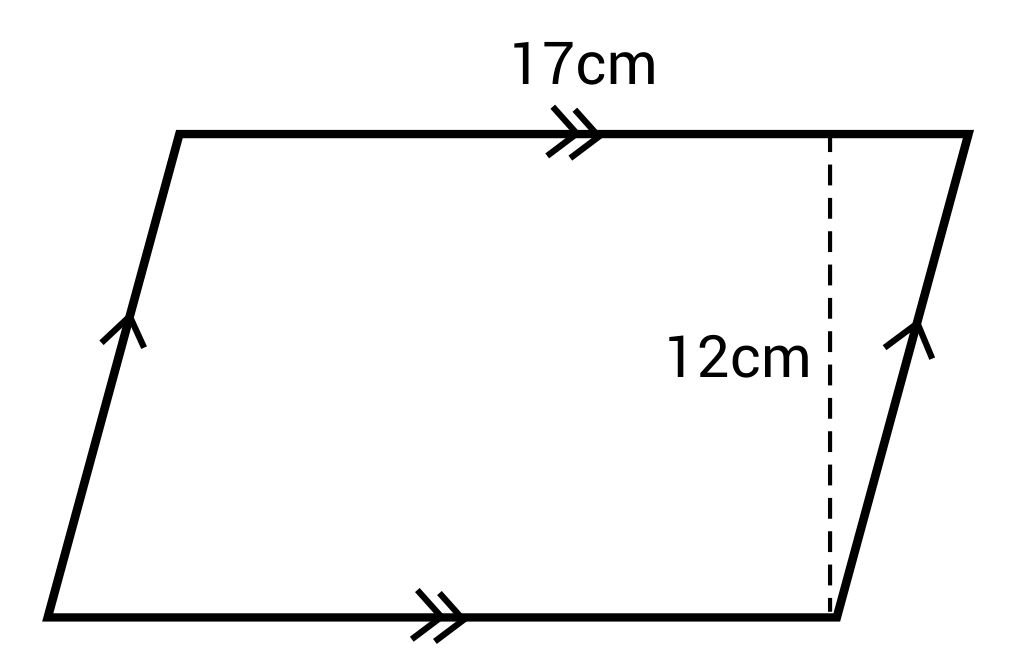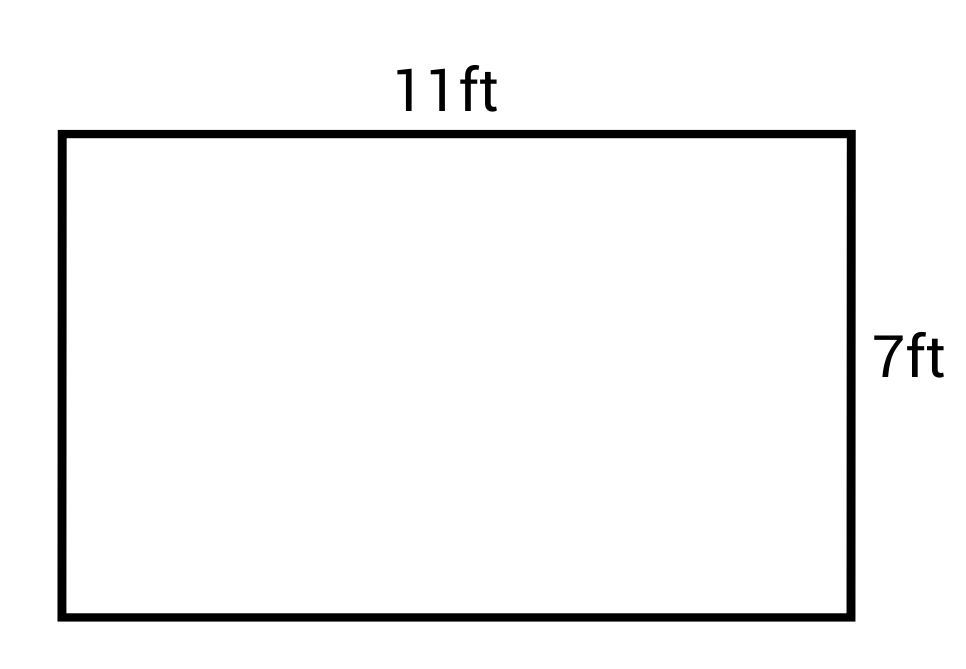# An Intro to Parallelograms

Welcome to this video lesson on common quadrilaterals.

Today, we will go over parallelograms, rectangles, and squares.

a) parallelogram = A parallelogram is a quadrilateral where all opposite sides are parallel and of equal length, and all opposite angles are equal.

To find the area of a parallelogram, multiply the length of of the sides, and multiply it by the height. In this case: x times h = area.

b) rectangle = A rectangle is a quadrilateral where all angles are equal to 90º, and all opposite sides are of equal length. The area of a rectangle is very easy to find, just like a parallelogram, height times width, in this case, x times y.

c) square = A square is a quadrilateral where all side lengths and angle measures are equal. Since all sides are equal, finding the area is simply a matter of multiplying any side by itself.

I hope that helps. Thanks for watching this video lesson, and until next time, happy studying.

## Practice Questions

Question #1:

Which of the following is not part of the definition of a parallelogram?

All angles are right angles

Opposite sides are parallel and of equal length

All opposite angles are equal

The correct answer is all angles are right angles. The definition of a parallelogram is, a quadrilateral where all opposite sides are parallel and of equal length, and all opposite angles are equal.

Question #2:

What is the area of this parallelogram?102 cm2

212 cm2

119 cm2

204 cm2

The correct answer is 204 cm2. The formula of a parallelogram is:
$$A=bh$$
The base (b) is 17 cm and the height (h) is 12 cm.
$$A=(170)(12)=204\text{ cm}^2$$
The area of this parallelogram is 204 cm2.

Question #3:

“A quadrilateral where all side lengths and angle measures are equal” is the definition of what shape?

Parallelogram

Rectangle

Square

Trapezoid

The correct answer is a square. This is the exact definition of a square. It has all equal sides and all equal angles.

Question #4:

What is the area of a square with a side length of 4 in?

24 in2

16 in2

8 in2

32 in2

The correct answer is 16 in2. The formula for area of a square is:
$$A=s^2$$
The length of the side (s) is 4 in.
$$A=(4)^2=16\text{ in}^2$$
The area of this square is 16 square inches.

Question #5:

What is the area of this rectangle?77 ft2

60 ft2

36 ft2

42 ft2

The correct answer is 77 ft2. The formula for area of a rectangle is:
$$A=lw$$
The length (l) is 17 ft and the width (w) is 7 ft.
$$A=(11)(7)=77\text{ ft}^2$$
The area of this rectangle is 77 square inches.

129981

by Mometrix Test Preparation | This Page Last Updated: July 19, 2023

Get Actionable Study Tips
Join our newsletter to get the study tips, test-taking strategies, and key insights that high-performing students use.
Get Actionable Study Tips
Join our newsletter to get the study tips, test-taking strategies, and key insights that high-performing students use!